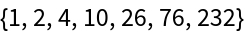Function Repository Resource:

# ParityPairings

List all possible partitions of a list into disjoint pairs

 ResourceFunction["ParityPairings"][items] lists all partitionings of items into disjoint pairs matching each single item once to any other or to itself.

## Details

If items has length n, each output partitioning has dimensions {k,2}, where n/2kn.
Each item from items appears exactly once as a member of a non-identical pair {l,m} or exactly twice as both members of an identical pair {m,m}.
When the items are chosen to be a range of numbers 1, 2, …, n, ResourceFunction["ParityPairings"] lists involutions of length n almost in Cycles notation, except that fixed indices are also listed as pairs {m,m}.

## Examples

### Basic Examples (2)

The only pairing of one item is the Identity:

 In:=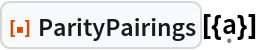Out=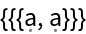Two items admit two distinct pairings:

 In:=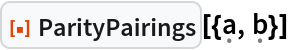Out=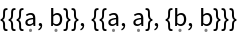Alternatively, items can also be entered as integers:

 In:=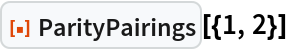Out=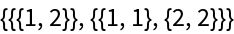### Properties and Relations (2)

Compare with the result of the resource function Involutions:

 In:=Out=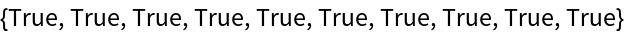Plot the relative time savings:

 In:=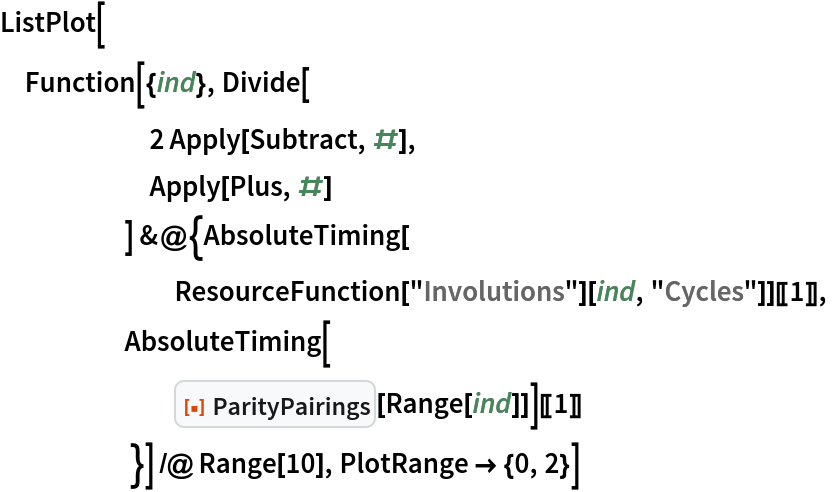Out=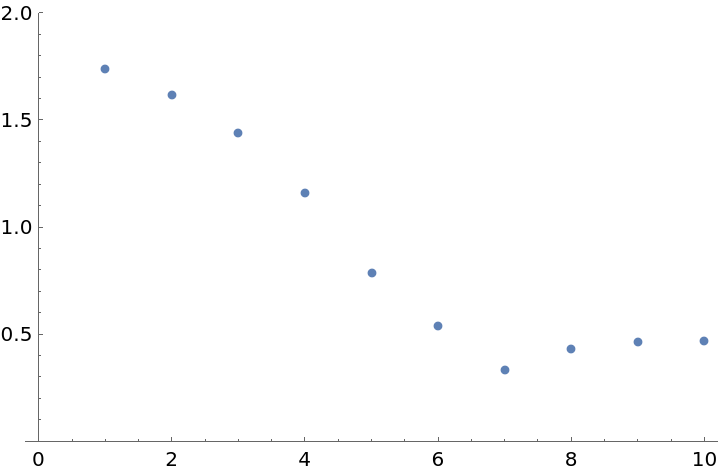### Neat Examples (1)

Count the number of distinct involutions on n letters (cf. OEIS A000085):

 In:=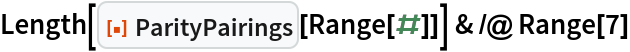Out=